### ReviewEcon.com

AP, IB, and College Microeconomic and Macroeconomic Principles

# Everything doing is worth doing well? Not so fast…

## Everything doing is worth doing well? Not so fast…

6/4/2020 Jacob Reed
Many people believe you should always do your very best work with everything you do. Those people are not economists. There are plenty of things people do and should do in this world that aren’t done very well and that is not only OK, it’s a great use of resources.

Below you will learn how economists believe choices should be made to get the most out of the resources we have.Marginal Analysis

The most repeated concept in all of Microeconomics is marginal analysis. Economists like to say decisions are made at the margin. That means most decisions are not all or nothing. Instead, they focus on incremental changes. A professional athlete, for example, isn’t usually deciding whether or not to train, rather they are deciding how long and how often to train. And with each additional minute of training, the athlete analyzes the costs and benefits of that additional minute.

Total Benefit vs Marginal Benefit

Another misconception many non-economists have is that people should work to maximize their total benefits.

Lets look at a decision regarding how long to study for your next exam as an example. Take a look at this chart showing grades you could earn with different amounts of studying.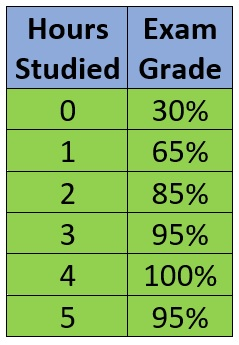If an economics student wanted to maximize their total benefit, they would study 4 hours. The total benefit of studying 4 hours is a grade of 100% on the exam. No other amount of studying would allow this student to get such a high grade?

So how many hours should you study if you are this student? 4 obviously…

Actually no; because decisions are made at the margin. Which means you must look at marginal costs and marginal benefits when making decisions.

Marginal is a term you will see repeated over and over again in microeconomics. Marginal simply means change in the total. In the case of marginal benefit for studying, that is the change in the total benefit for the next hour studied.

Take a look at the new chart below. There is a new column for the marginal benefit of each hour studied and percentages have been changed to a dollar value (we’ll assume each percentage point is worth \$1 to you) to make comparisons to cost easier later on. The marginal benefit is the change in total benefit for that hour. The marginal benefit for the first hour studied is 35% (65% – 30%), while the marginal benefit for the 3rd hour is 10% (95% – 85%).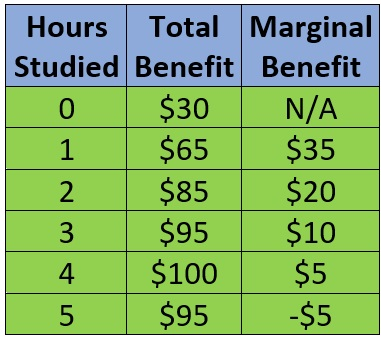Marginal benefits, generally decrease with each unit. The more you do anything, the marginal benefit of the activity tends to decrease. On a graph, marginal benefit is illustrated as a downward sloping curve.

Total Cost vs Marginal Cost

Of course, benefits aren’t all we should be looking at. We must also look at the costs (marginal costs) associated with studying. With each hour studied your time becomes more and more precious to you. As a result, marginal costs generally increase with each additional unit. If we quantify that cost in dollars, we might see a chart that looks like this: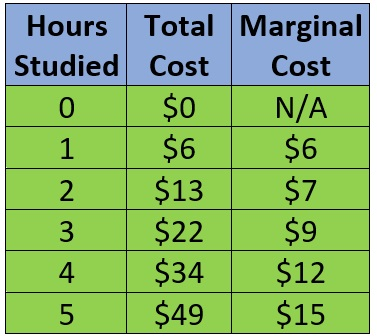Marginal costs, generally increase with each unit. The more you do anything, the marginal cost of the activity tends to increase. On a graph, marginal cost is illustrated as an upward sloping curve.

What is benefit maximizing behavior?

A person maximizes benefit when they keep doing something as long as the marginal benefit (MB) is greater than or equal to the marginal cost (MC). In the example above, the first 3 hours of studying have a greater marginal benefit than marginal cost. Studying for those hours will increase your net benefit (total benefit – total cost). Hours 4 and 5 of studying have a greater marginal cost than marginal benefit. So, studying those hours would make you worse off and decrease your net benefit. Benefit maximzation is found where MB=MC. If they are never equal, as in the example above, the largest quantity where MB>MC will maximize benefit.

On a graph, Marginal cost curves slope upward and marginal benefit curves slope downward and the benefit maximizing quantity is found where the marginal benefit and marginal cost curves intersect. At lower quantities, the marginal benefit is greater than the marginal cost and net benefit would increase with greater quantities. At high quantities, the marginal benefit is less than the marginal cost and net benefit would increase with lower quantities.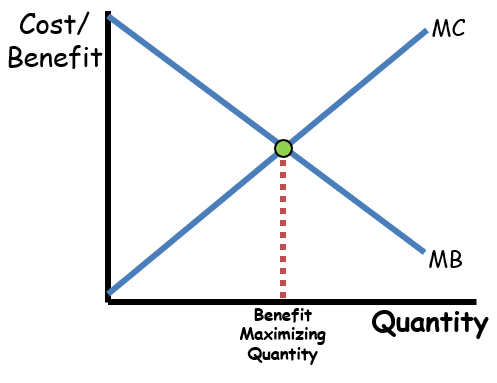This concept of benefit maximization where marginal benefit equals marginal cost, is the most repeated concept in all of microeconomics. As you move through this course the term used for marginal benefit will change. For consumers the term marginal utility (satisfaction gained by consuming more units of a good) is often used in lieu of marginal benefit. For firms selling products, the marginal benefit is called marginal revenue and for firms buying resources (like labor), it’s called marginal revenue product. Despite the different vocabulary you will see, the concept remains the same. Benefit maximization is found where marginal benefit equals marginal cost.

Whether it’s a business deciding how much to produce, an athlete decided how much to exercise, or the government deciding how much pollution to clean up, the benefit maximizing choice is found where marginal benefit equals marginal cost.

So make sure you study economics, as long as the marginal benefit of studying exceeds the marginal cost and take a break as soon as they are equal. You might do better on your next exam if you studied longer, but it just wouldn’t be worth it. 😊

How does marginal analysis apply to consumer behavior?
Marginal analysis can be applied to consumer behavior by understanding the concept of Diminishing Marginal Utility. This is a microeconomic concept that tells us the benefit (utility) gained by consuming more of a good or service decreases with each unit consumed.  So if Jenny eats 1 cheeseburger, she might gain 100 utils (units of satisfaction), eating a second cheeseburger might be worth 50 utils to her, a third worth 10, and a fourth worth 1 util. While her total utility increases with each cheeseburger consumed (100, 150, 160, then 161), the marginal utility (additional benefit) decreases with each cheeseburger consumed. As a result when prices rise, the fewer units are worth buying for consumers.

Marginal Utility per Dollar is a calculation of a value to price ratio for the consumption of an additional unit of a product. The formula is Marginal Utility divided by Price (MU/P). Using the cheeseburger example above, if each cheese burger costs \$5, the Marginal utility per dollar for the second cheeseburger would be 10 utils per dollar (50/\$5).

Utility Maximization occurs when a consumer adjusts their spending to maximize their total utility within their given income limitations. Utility maximization occurs when the marginal utility per dollar for all the goods you purchase are equal (MUx/Px=MUy/Py). To simplify, let’s assume Jenny only buys 2 different goods; cheeseburgers and soda.  The 2 charts below show her the utility per dollar for both goods.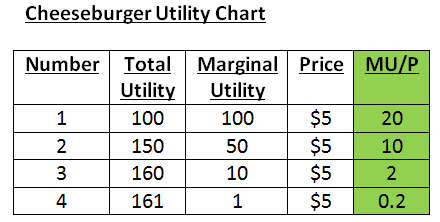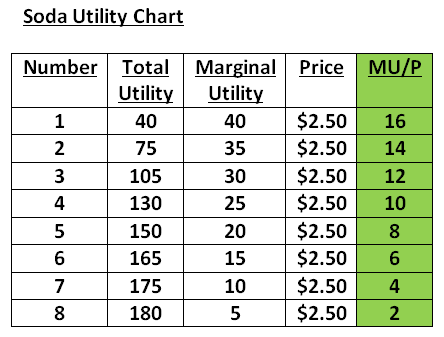​If Jenny has \$20 to spend, she would maximize her utility by purchasing 4 sodas and 2 cheeseburgers.  There, the marginal utility per dollar (MU/P) for cheeseburgers is 10 and the marginal utility per dollar for sodas is 10. That would give her a total utility of 280 utils. Given the fact she only has \$20, she cannot get more utility than that. She has maximized her utility.

If Jenny’s current consumption was 6 sodas and 1 cheeseburger the marginal utililty per dollar for cheeseburgers (20) would be greater than the marginal utility per dollar for sodas (6).  When the marginal utility per dollar for both goods is not equal, a consumer would increase total utility by consuming less of the item with a low marginal utility per dollar and more of the item with a high marginal utility per dollar. In other words, the consumer should buy more of the item giving her a better deal and less of the item giving her a worse deal.

**Note: you could see questions exactly like this where total benefit or marginal benefit are used instead of total utility or marginal utility. Since benefit and utility are essentially synonyms, you will answer the question exact same way.

This method of utility maximization is very similar to profit maximizing combinations and cost minimizing combinations of resources. Producers just calculate the marginal product per dollar or marginal revenue product per dollar for productive resources instead.

Multiple Choice Connections:
2012 Released AP Microeconomics Exam Questions: 49, 51

Up Next:
Review Game: Best Combinations review (1st 5 questions)
Content Review Page: Cost Curves, Revenue Curves, and Profit

Other recommended resourcesJodi Econ Girl